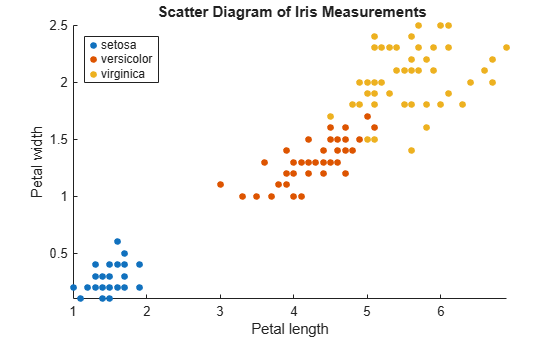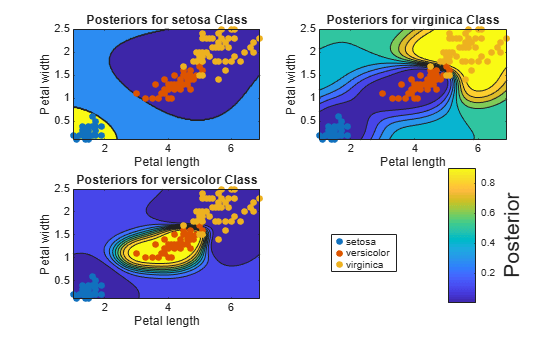# fitPosterior

Fit posterior probabilities for support vector machine (SVM) classifier

## Syntax

ScoreSVMModel = fitPosterior(SVMModel)
[ScoreSVMModel,ScoreTransform] = fitPosterior(SVMModel)
[ScoreSVMModel,ScoreTransform] = fitPosterior(SVMModel,Name,Value)

## Description

example

ScoreSVMModel = fitPosterior(SVMModel) returns a trained support vector machine (SVM) classifier ScoreSVMModel containing the optimal score-to-posterior-probability transformation function for two-class learning. For more details, see Algorithms.

example

[ScoreSVMModel,ScoreTransform] = fitPosterior(SVMModel) additionally returns the optimal score-to-posterior-probability transformation function parameters.

example

[ScoreSVMModel,ScoreTransform] = fitPosterior(SVMModel,Name,Value) uses additional options specified by one or more name-value pair arguments. For example, you can specify the number of folds or the holdout sample proportion.

## Examples

collapse all

Load the ionosphere data set.

load ionosphere

Train an SVM classifier. Standardize the data and specify that 'g' is the positive class.

SVMModel = fitcsvm(X,Y,'ClassNames',{'b','g'},'Standardize',true);

SVMModel is a ClassificationSVM classifier.

Fit the optimal score-to-posterior-probability transformation function.

rng(1); % For reproducibility ScoreSVMModel = fitPosterior(SVMModel)
ScoreSVMModel = ClassificationSVM ResponseName: 'Y' CategoricalPredictors: [] ClassNames: {'b' 'g'} ScoreTransform: '@(S)sigmoid(S,-9.479889e-01,-1.220433e-01)' NumObservations: 351 Alpha: [90x1 double] Bias: -0.1342 KernelParameters: [1x1 struct] Mu: [1x34 double] Sigma: [1x34 double] BoxConstraints: [351x1 double] ConvergenceInfo: [1x1 struct] IsSupportVector: [351x1 logical] Solver: 'SMO' Properties, Methods 

Because the classes are inseparable, the score transformation function (ScoreSVMModel.ScoreTransform) is the sigmoid function.

Estimate scores and positive class posterior probabilities for the training data. Display the results for the first 10 observations.

[label,scores] = resubPredict(SVMModel); [~,postProbs] = resubPredict(ScoreSVMModel); table(Y(1:10),label(1:10),scores(1:10,2),postProbs(1:10,2),'VariableNames',... {'TrueLabel','PredictedLabel','Score','PosteriorProbability'})
ans=10×4 table TrueLabel PredictedLabel Score PosteriorProbability _________ ______________ _______ ____________________ {'g'} {'g'} 1.4861 0.82213 {'b'} {'b'} -1.0002 0.30446 {'g'} {'g'} 1.8684 0.86913 {'b'} {'b'} -2.6459 0.084225 {'g'} {'g'} 1.2805 0.79183 {'b'} {'b'} -1.4615 0.22039 {'g'} {'g'} 2.1672 0.89812 {'b'} {'b'} -5.7081 0.0050204 {'g'} {'g'} 2.4796 0.9222 {'b'} {'b'} -2.7809 0.074869 

Train a multiclass SVM classifier through the process of one-versus-all (OVA) classification, and then plot probability contours for each class. To implement OVA directly, see fitcecoc.

Load Fisher's iris data set. Use the petal lengths and widths as the predictor data.

load fisheriris X = meas(:,3:4); Y = species;

Examine a scatter plot of the data.

figure gscatter(X(:,1),X(:,2),Y); title('{\bf Scatter Diagram of Iris Measurements}'); xlabel('Petal length'); ylabel('Petal width'); legend('Location','Northwest'); axis tightTrain three binary SVM classifiers that separate each type of iris from the others. Assume that a radial basis function is an appropriate kernel for each, and allow the algorithm to choose a kernel scale. Define the class order.

classNames = {'setosa'; 'virginica'; 'versicolor'}; numClasses = size(classNames,1); inds = cell(3,1); % Preallocation SVMModel = cell(3,1); rng(1); % For reproducibility for j = 1:numClasses inds{j} = strcmp(Y,classNames{j}); % OVA classification SVMModel{j} = fitcsvm(X,inds{j},'ClassNames',[false true],... 'Standardize',true,'KernelFunction','rbf','KernelScale','auto'); end

fitcsvm uses a heuristic procedure that involves subsampling to compute the value of the kernel scale.

Fit the optimal score-to-posterior-probability transformation function for each classifier.

for j = 1:numClasses SVMModel{j} = fitPosterior(SVMModel{j}); end
Warning: Classes are perfectly separated. The optimal score-to-posterior transformation is a step function. 

Define a grid to plot the posterior probability contours. Estimate the posterior probabilities over the grid for each classifier.

d = 0.02; [x1Grid,x2Grid] = meshgrid(min(X(:,1)):d:max(X(:,1)),... min(X(:,2)):d:max(X(:,2))); xGrid = [x1Grid(:),x2Grid(:)]; posterior = cell(3,1); for j = 1:numClasses [~,posterior{j}] = predict(SVMModel{j},xGrid); end

For each SVM classifier, plot the posterior probability contour under the scatter plot of the data.

figure h = zeros(numClasses + 1,1); % Preallocation for graphics handles for j = 1:numClasses subplot(2,2,j) contourf(x1Grid,x2Grid,reshape(posterior{j}(:,2),size(x1Grid,1),size(x1Grid,2))); hold on h(1:numClasses) = gscatter(X(:,1),X(:,2),Y); title(sprintf('Posteriors for %s Class',classNames{j})); xlabel('Petal length'); ylabel('Petal width'); legend off axis tight hold off end h(numClasses + 1) = colorbar('Location','EastOutside',... 'Position',[[0.8,0.1,0.05,0.4]]); set(get(h(numClasses + 1),'YLabel'),'String','Posterior','FontSize',16); legend(h(1:numClasses),'Location',[0.6,0.2,0.1,0.1]);Estimate the score-to-posterior-probability transformation function after training an SVM classifier. Use cross-validation during the estimation to reduce bias, and compare the run times for 10-fold cross-validation and holdout cross-validation.

Load the ionosphere data set.

load ionosphere

Train an SVM classifier. Standardize the data and specify that 'g' is the positive class.

SVMModel = fitcsvm(X,Y,'ClassNames',{'b','g'},'Standardize',true);

SVMModel is a ClassificationSVM classifier.

Fit the optimal score-to-posterior-probability transformation function. Compare the run times from using 10-fold cross-validation (the default) and a 10% holdout test sample.

rng(1); % For reproducibility tic; % Start the stopwatch SVMModel_10FCV = fitPosterior(SVMModel); toc % Stop the stopwatch and display the run time
Elapsed time is 0.940837 seconds. 
tic; SVMModel_HO = fitPosterior(SVMModel,'Holdout',0.10); toc
Elapsed time is 0.208140 seconds. 

Although both run times are short because the data set is relatively small, SVMModel_HO fits the score transformation function much faster than SVMModel_10FCV. You can specify holdout cross-validation (instead of the default 10-fold cross validation) to reduce run time for larger data sets.

## Input Arguments

collapse all

Full, trained SVM classifier, specified as a ClassificationSVM model trained with fitcsvm.

### Name-Value Pair Arguments

Specify optional comma-separated pairs of Name,Value arguments. Name is the argument name and Value is the corresponding value. Name must appear inside quotes. You can specify several name and value pair arguments in any order as Name1,Value1,...,NameN,ValueN.

Example: fitPosterior(SVMModel,'KFold',5) uses five folds in a cross-validated model.

Cross-validation partition used to compute the transformation function, specified as the comma-separated pair consisting of 'CVPartition' and a cvpartition partition object as created by cvpartition. You can use only one of these four options at a time for creating a cross-validated model: 'KFold', 'Holdout', 'Leaveout', or 'CVPartition'.

The crossval name-value pair argument of fitcsvm splits the data into subsets using cvpartition.

Example: Suppose you create a random partition for 5-fold cross-validation on 500 observations by using cvp = cvpartition(500,'KFold',5). Then, you can specify the cross-validated model by using 'CVPartition',cvp.

Fraction of the data for holdout validation used to compute the transformation function, specified as the comma-separated pair consisting of 'Holdout' and a scalar value in the range (0,1). Holdout validation tests the specified fraction of the data and uses the remaining data for training.

You can use only one of these four options at a time for creating a cross-validated model: 'KFold', 'Holdout', 'Leaveout', or 'CVPartition'.

Example: 'Holdout',0.1

Data Types: double | single

Number of folds to use when computing the transformation function, specified as the comma-separated pair consisting of 'KFold' and a positive integer value greater than 1.

You can use only one of these four options at a time for creating a cross-validated model: 'KFold', 'Holdout', 'Leaveout', or 'CVPartition'.

Example: 'KFold',8

Data Types: single | double

Leave-one-out cross-validation flag indicating whether to use leave-one-out cross-validation to compute the transformation function, specified as the comma-separated pair consisting of 'Leaveout' and 'on' or 'off'. Use leave-one-out cross-validation by specifying 'Leaveout','on'.

You can use only one of these four options at a time for creating a cross-validated model: 'KFold', 'Holdout', 'Leaveout', or 'CVPartition'.

Example: 'Leaveout','on'

## Output Arguments

collapse all

Trained SVM classifier, returned as a ClassificationSVM classifier. The trained classifier contains the estimated score-to-posterior-probability transformation function.

To estimate posterior probabilities for the training set observations, pass ScoreSVMModel to resubPredict.

To estimate posterior probabilities for new observations, pass the new observations and ScoreSVMModel to predict.

Optimal score-to-posterior-probability transformation function parameters, returned as a structure array.

• If the value of the Type field of ScoreTransform is sigmoid, then ScoreTransform also has these fields:

• Slope: The value of A in the sigmoid function

• Intercept: The value of B in the sigmoid function

• If the value of the Type field of ScoreTransform is step, then ScoreTransform also has these fields:

• PositiveClassProbability: The value of π in the step function. This value represents the probability that an observation is in the positive class or the posterior probability that an observation is in the positive class given that its score is in the interval (LowerBound,UpperBound).

• LowerBound: The value $\underset{{y}_{n}=-1}{\mathrm{max}}{s}_{n}$ in the step function. This value represents the lower bound of the score interval that assigns observations with scores in the interval the posterior probability of being in the positive class PositiveClassProbability. Any observation with a score less than LowerBound has the posterior probability of being in the positive class equal to 0.

• UpperBound: The value $\underset{{y}_{n}=+1}{\mathrm{min}}{s}_{n}$ in the step function. This value represents the upper bound of the score interval that assigns observations with scores in the interval the posterior probability of being in the positive class PositiveClassProbability. Any observation with a score greater than UpperBound has the posterior probability of being in the positive class equal to 1.

• If the value of the Type field of ScoreTransform is constant, then ScoreTransform.PredictedClass contains the name of the class prediction.

This result is the same as SVMModel.ClassNames. The posterior probability of an observation being in ScoreTransform.PredictedClass is always 1.

collapse all

### Sigmoid Function

The sigmoid function that maps score sj corresponding to observation j to the positive class posterior probability is

$P\left({s}_{j}\right)=\frac{1}{1+\mathrm{exp}\left(A{s}_{j}+B\right)}.$

If the value of the Type field of ScoreTransform is sigmoid, then parameters A and B correspond to the fields Scale and Intercept of ScoreTransform, respectively.

### Step Function

The step function that maps score sj corresponding to observation j to the positive class posterior probability is

$P\left({s}_{j}\right)=\left\{\begin{array}{l}\begin{array}{cc}0;& s<\underset{{y}_{k}=-1}{\mathrm{max}}{s}_{k}\end{array}\\ \begin{array}{cc}\pi ;& \underset{{y}_{k}=-1}{\mathrm{max}}{s}_{k}\le {s}_{j}\le \underset{{y}_{k}=+1}{\mathrm{min}}{s}_{k}\end{array}\\ \begin{array}{cc}1;& {s}_{j}>\underset{{y}_{k}=+1}{\mathrm{min}}{s}_{k}\end{array}\end{array},$

where:

• sj is the score of observation j.

• +1 and –1 denote the positive and negative classes, respectively.

• π is the prior probability that an observation is in the positive class.

If the value of the Type field of ScoreTransform is step, then the quantities $\underset{{y}_{k}=-1}{\mathrm{max}}{s}_{k}$ and $\underset{{y}_{k}=+1}{\mathrm{min}}{s}_{k}$ correspond to the fields LowerBound and UpperBound of ScoreTransform, respectively.

### Constant Function

The constant function maps all scores in a sample to posterior probabilities 1 or 0.

If all observations have posterior probability 1, then they are expected to come from the positive class.

If all observations have posterior probability 0, then they are not expected to come from the positive class.

## Tips

• This process describes one way to predict positive class posterior probabilities.

1. Train an SVM classifier by passing the data to fitcsvm. The result is a trained SVM classifier, such as SVMModel, that stores the data. The software sets the score transformation function property (SVMModel.ScoreTransformation) to none.

2. Pass the trained SVM classifier SVMModel to fitSVMPosterior or fitPosterior. The result, such as, ScoreSVMModel, is the same trained SVM classifier as SVMModel, except the software sets ScoreSVMModel.ScoreTransformation to the optimal score transformation function.

3. Pass the predictor data matrix and the trained SVM classifier containing the optimal score transformation function (ScoreSVMModel) to predict. The second column in the second output argument of predict stores the positive class posterior probabilities corresponding to each row of the predictor data matrix.

If you skip step 2, then predict returns the positive class score rather than the positive class posterior probability.

• After fitting posterior probabilities, you can generate C/C++ code that predicts labels for new data. Generating C/C++ code requires MATLAB® Coder™. For details, see Introduction to Code Generation.

## Algorithms

The software fits the appropriate score-to-posterior-probability transformation function by using the SVM classifier SVMModel and by conducting 10-fold cross-validation using the stored predictor data (SVMModel.X) and the class labels (SVMModel.Y), as outlined in . The transformation function computes the posterior probability that an observation is classified into the positive class (SVMModel.Classnames(2)).

• If the classes are inseparable, then the transformation function is the sigmoid function.

• If the classes are perfectly separable, then the transformation function is the step function.

• In two-class learning, if one of the two classes has a relative frequency of 0, then the transformation function is the constant function. The fitPosterior function is not appropriate for one-class learning.

• The software stores the optimal score-to-posterior-probability transformation function in ScoreSVMModel.ScoreTransform.

If you re-estimate the score-to-posterior-probability transformation function, that is, if you pass an SVM classifier to fitPosterior or fitSVMPosterior and its ScoreTransform property is not none, then the software:

• Displays a warning

• Resets the original transformation function to 'none' before estimating the new one

## Alternative Functionality

You can also fit the posterior probability function by using fitSVMPosterior. This function is similar to fitPosterior, except it is more broad because it accepts a wider range of SVM classifier types.

 Platt, J. “Probabilistic outputs for support vector machines and comparisons to regularized likelihood methods.” Advances in Large Margin Classifiers. Cambridge, MA: The MIT Press, 2000, pp. 61–74.